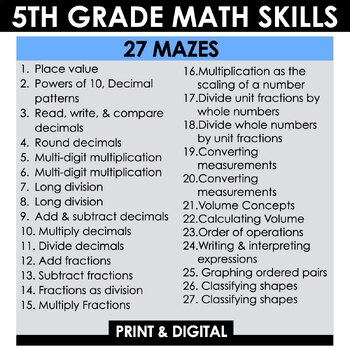DID YOU KNOW:
Seamlessly assign resources as digital activities

Learn how in 5 minutes with a tutorial resource. Try it Now

Learn More# 5th Grade Math Mazes - 5th Grade Math Test Prep - Fun Math Review Bundle5th
Subjects
Standards
Resource Type
Formats Included
• Zip
Pages
27 mazes
\$21.60
Bundle
List Price:
\$27.00
You Save:
\$5.40
\$21.60
Bundle
List Price:
\$27.00
You Save:
\$5.40This bundle contains one or more resources with Google apps (e.g. docs, slides, etc.).

#### Also included in

1. Review 3rd, 4th, and 5th grade math standards with these FUN Math Mazes. This mega bundle includes 80 mazes that will keep students engaged! Use during math stations, math homework, or math test prep.Save 30% when you purchase this bundle. If you purchase individually, this bundle has a value of \$80
\$56.00
\$80.00
Save \$24.00

### Description

Review 5th grade math standards with these FUN Math Mazes. This complete bundle includes 27 mazes that will keep students engaged! Use during math stations, math homework, or math test prep.

Save 20% when you purchase this bundle. If you purchase individually, this bundle has a value of \$27.

Included:

Set 1:

Maze 1 - Place value

Maze 2 - Powers of 10, Decimal patterns

Maze 3 - Read, write, and compare decimals to the thousandths place

Maze 4 - Round decimals

Maze 5 - Multiply 3-digit numbers by 2-digit numbers, Multiply 3-digit numbers by 3-digit numbers

Maze 6 - Fluently multiply multi-digit whole numbers

Maze 7 - Divide 3-digit numbers by 2 digit numbers

Maze 8 - Divide 4-digit numbers by 2-digit numbers

Maze 9 - Add and subtract decimals

Maze 10 - Multiply decimals to the hundredths place

Maze 11 - Divide decimals to the hundredths place

Set 2:

Maze 12 - Add fractions with unlike denominators (including mixed numbers)

Maze 13 - Subtract fractions with unlike denominators (including mixed numbers)

Maze 14 - Interpret a fraction as division of the numerator by the denominator

Maze 15 - Multiply a fraction or whole number by a fraction

Maze 16 - Multiplication as the scaling of a number

Maze 17 - Divide unit fractions by whole numbers

Maze 18 - Divide whole numbers by unit fractions

Set 3:

Maze 19 - Converting measurements

Maze 20 - Converting measurements

Maze 21 - Volume (counting unit cubes)

Maze 22 - Volume (using the formulas V = l x w x h and V = b x h)

Set 4:

Maze 23 - Order of operations

Maze 24 - Writing and interpreting expressions

Maze 25 - Graphing ordered pairs

Maze 26 - Classifying shapes

Maze 27 - Classifying shapes

F.A.Q.

Are the mazes aligned to the CCSS? Yes. Not a common core state? I include a version without the standards.

Are the mazes editable? No.

Are the mazes available in digital format? Yes. I include a Google Slides version with moveable arrows.

>> More Math Mazes <<

PAPERLESS Math Resources

Total Pages
27 mazes
Included
Teaching Duration
N/A
Report this Resource to TpT
Reported resources will be reviewed by our team. Report this resource to let us know if this resource violates TpT’s content guidelines.

### Standards

to see state-specific standards (only available in the US).
Classify two-dimensional figures in a hierarchy based on properties.
Understand that attributes belonging to a category of two-dimensional figures also belong to all subcategories of that category. For example, all rectangles have four right angles and squares are rectangles, so all squares have four right angles.
Use a pair of perpendicular number lines, called axes, to define a coordinate system, with the intersection of the lines (the origin) arranged to coincide with the 0 on each line and a given point in the plane located by using an ordered pair of numbers, called its coordinates. Understand that the first number indicates how far to travel from the origin in the direction of one axis, and the second number indicates how far to travel in the direction of the second axis, with the convention that the names of the two axes and the coordinates correspond (e.g., 𝘹-axis and 𝘹-coordinate, 𝘺-axis and 𝘺-coordinate).
Interpret a fraction as division of the numerator by the denominator (𝘢/𝘣 = 𝘢 ÷ 𝘣). Solve word problems involving division of whole numbers leading to answers in the form of fractions or mixed numbers, e.g., by using visual fraction models or equations to represent the problem. For example, interpret 3/4 as the result of dividing 3 by 4, noting that 3/4 multiplied by 4 equals 3, and that when 3 wholes are shared equally among 4 people each person has a share of size 3/4. If 9 people want to share a 50-pound sack of rice equally by weight, how many pounds of rice should each person get? Between what two whole numbers does your answer lie?
Add and subtract fractions with unlike denominators (including mixed numbers) by replacing given fractions with equivalent fractions in such a way as to produce an equivalent sum or difference of fractions with like denominators. For example, 2/3 + 5/4 = 8/12 + 15/12 = 23/12. (In general, 𝘢/𝘣 + 𝘤/𝘥 = (𝘢𝘥 + 𝘣𝘤)/𝘣𝘥.)